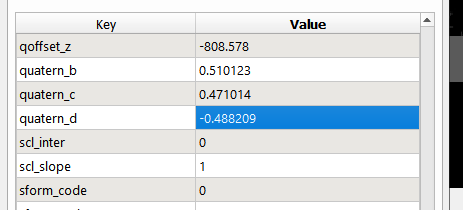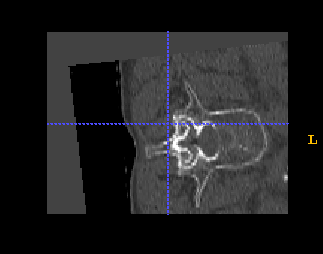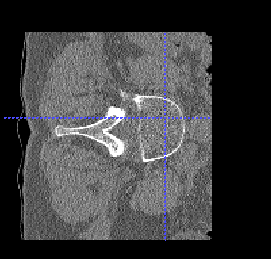# Rotating an Oblique Image

I have been trying to rotate an image that has quaterion coordinates and is in an oblique reference frame, but I am not entirely sure how this is done. When I apply the same rotations (two rotations about the x and y axes) to a non-oblique image, it ends up in the proper orientation. However, when trying with the image with the quaterion rotations, it ends up slightly off tilt (almost like a picture off center in a picture frame). The images in question are 3D images and I have been trying both the VersorTransform and the Eurler3DTransform for the rotations, but with no success with the oblique images.

Welcome to the community Geoff!

1 Like

Sure thing. Here is the snippet of code that I am using. Essentially I am rotating in two axis, and I have confirmed this does work when the images are not in an oblique view. The images are starting in ASL orientation and I am rotating them into SLA orientation. Below are the images with the quaternion values taken all screen shot from ITK-Snap. The left image is the one with the quaterion angles and the right is the one that worked properly.

``````    euler = sitk.Euler3DTransform()
euler.SetComputeZYX(True)
a = np.array([[1., 0, 0],
[0, 0, 1.],
[0, -1., 0]])

b = np.array([[0, 1., 0],
[-1., 0, 0],
[0, 0, 1.]])

c = np.matmul(b, a)

euler.SetMatrix(tuple(c.flatten()))
euler.SetCenter(img_resampled.GetOrigin())

old_direction = np.array(img.GetDirection()).reshape(3, 3)
new_direction = np.matmul(c, old_direction)

image_shape = img.GetSize()

resample = sitk.ResampleImageFilter()
resample.SetReferenceImage(img)

resample.SetSize([image_shape, image_shape, image_shape])
resample.SetOutputDirection(tuple(new_direction.flatten()))

resample.SetInterpolator(sitk.sitkLinear)
resample.SetTransform(euler)

img_resampled = resample.Execute(img)
``````